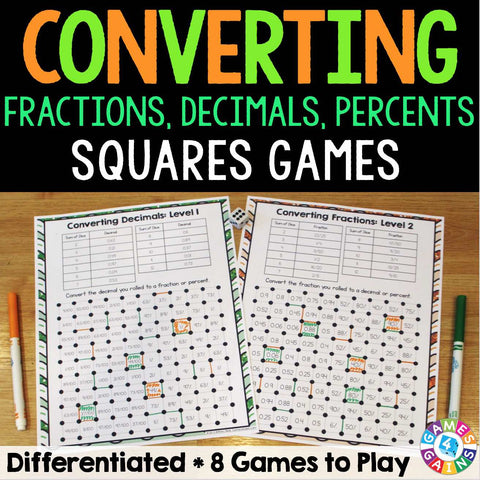## Fractions, Decimals, and Percents 'Squares' Game

• \$350

Converting Fractions, Decimals, and Percents "Squares" game contains 8 fun and engaging games to help students practice converting various fractions, decimals, and percents.

In these games, students will practice:
• Converting fractions to decimals and percents
• Converting decimals to fractions and percents
• Converting percents to fractions and decimals
• BONUS: Converting ratios to decimals and percents

Each skill listed above (except for the bonus) includes more than one level of play. This means that you can differentiate the games for each student!

These Converting Fractions, Decimals, and Percents "Squares" games are so simple to use, and take a minimal amount of prep. Simply print out the game sheet, grab 2 dice and a few markers, and you'll be ready to go!

To play, students will roll the dice to find the fraction, decimal, or percent to convert. Then, students draw a line between two of the dots surrounding the answer. When a student's line forms a complete square, he/she gets to capture that square. The student with the most squares at the end of the game is the winner!

Included with this Converting Fractions, Decimals, and Percents "Squares" Game:
Instructions for play
8 "squares" game boards